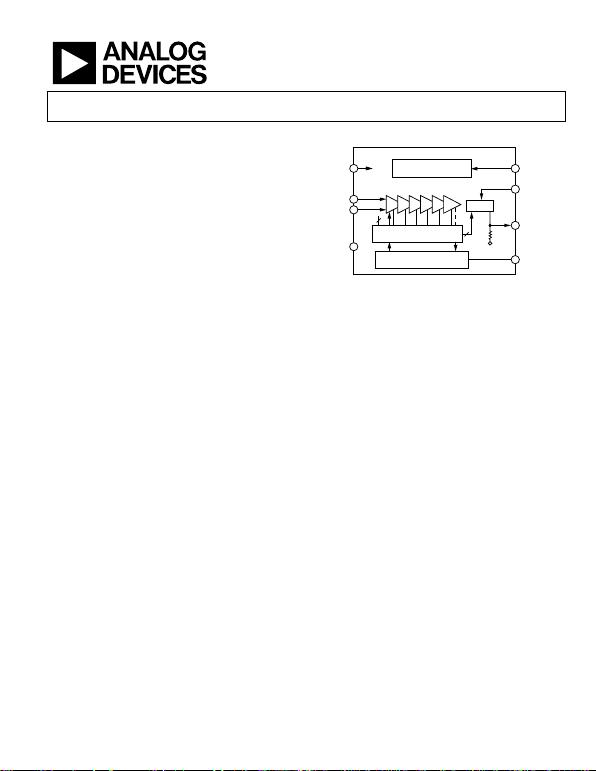Low Cost, DC to 500 MHz, 92 dB
Logarithmic Amplifier
Rev. D
Information furnished by Analog Devices is believed to be accurate and reliable. However, no
responsibility is assumed by Analog Devices for its use, nor for any infringements of patents or other
rights of third parties that may result from its use. Specifications subject to change without notice. No
license is granted by implication or otherwise under any patent or patent rights of Analog Devices.
One Technology Way, P.O. Box 9106, Norwood, MA 02062-9106, U.S.A.
Tel: 781.329.4700 www.analog.com
FEATURES
Complete multistage logarithmic amplifier
92 dB dynamic range: –75 dBm to +17 dBm
to –90 dBm using matching network
Single supply of 2.7 V minimum at 7.5 mA typical
DC to 500 MHz operation, ±1 dB linearity
Slope of 25 mV/dB, intercept of −84 dBm
Highly stable scaling over temperature
Fully differential dc-coupled signal path
100 ns power-up time, 150 A sleep current
APPLICATIONS
Conversion of signal level to decibel form
Transmitter antenna power measurement
Low cost radar and sonar signal processing
Network and spectrum analyzers (to 120 dB)
Signal level determination down to 20 Hz
True decibel ac mode for multimeters
FUNCTIONAL BLOCK DIAGRAM
BAND GAP REFERENCE
AND BIASING
SIX 14.3dB 900MHz
AMPLIFIER STAGES
MIRROR
INPUT-OFFSET
COMPENSATION LOOP
COM
INM
INP
ENBVPS
INT
OUT
OFS
7.5mA
1.1k
3
2
2µA
/dB
12.5k
COM
NINE DETECTOR CELLS
SPACED 14.3dB
–INP
+INP
8
1
2
7
5
6
4
3
0
1082-001
Figure 1.
GENERAL DESCRIPTION
an 8-lead (SOIC_N) package. It is a complete 500 MHz monolithic
demodulating logarithmic amplifier based on the progressive
compression (successive detection) technique, providing a
dynamic range of 92 dB to ±3 dB law-conformance and 88 dB
to a tight ±1 dB error bound at all frequencies up to 100 MHz.
It is extremely stable and easy to use, requiring no significant
external components. A single-supply voltage of 2.7 V to 5.5 V
at 7.5 mA is needed, corresponding to an unprecedented power
consumption of only 22.5 mW at 3 V. A fast acting CMOS-
compatible control pin can disable the AD8307 to a standby
current of less than 150 A.
Each of the cascaded amplifier/limiter cells has a small signal
gain of 14.3 dB, with a −3 dB bandwidth of 900 MHz. The input
is fully differential and at a moderately high impedance (1.1 k
dynamic range extending from approximately −75 dBm (where
dBm refers to a 50  source, that is, a sine amplitude of about
±56 V) up to +17 dBm (a sine amplitude of ±2.2 V). A simple
input matching network can lower this range to –88 dBm to
+3 dBm. The logarithmic linearity is typically within ±0.3 dB up
to 100 MHz over the central portion of this range, and degrades
only slightly at 500 MHz. There is no minimum frequency limit.
The AD8307 can be used at audio frequencies of 20 Hz or lower.
The output is a voltage scaled 25 mV/dB, generated by a current
of nominally 2 µA/dB through an internal 12.5 kΩ resistor. This
voltage varies from 0.25 V at an input of −74 dBm (that is, the
ac intercept is at −84 dBm, a 20 µV rms sine input), up to 2.5 V
for an input of +16 dBm. This slope and intercept can be trimmed
using external adjustments. Using a 2.7 V supply, the output
scaling can be lowered, for example to 15 mV/dB, to permit
utilization of the full dynamic range.
The AD8307 exhibits excellent supply insensitivity and temperature
stability of the scaling parameters. The unique combination of
low cost, small size, low power consumption, high accuracy and
stability, very high dynamic range, and a frequency range
encompassing audio through IF to UHF makes this product
useful in numerous applications requiring the reduction of a
signal to its decibel equivalent.
The AD8307 operates over the industrial temperature range of
PDIP packages.Rev. D | Page 2 of 24
Features .............................................................................................. 1
Applications ....................................................................................... 1
Functional Block Diagram .............................................................. 1
General Description ......................................................................... 1
Revision History ............................................................................... 2
Specifications ..................................................................................... 3
Absolute Maximum Ratings ............................................................ 4
ESD Caution .................................................................................. 4
Pin Configuration and Function Descriptions ............................. 5
Typical Performance Characteristics ............................................. 6
Log Amp Theory .............................................................................. 9
Progressive Compression .......................................................... 10
Demodulating Log Amps .......................................................... 11
Intercept Calibration .................................................................. 12
Offset Control ............................................................................. 12
Extension of Range ..................................................................... 13
Interfaces .......................................................................................... 14
Enable Interface .......................................................................... 14
Input Interface ............................................................................ 14
Offset Interface ........................................................................... 15
Output Interface ......................................................................... 15
Theory of Operation ...................................................................... 17
Basic Connections ...................................................................... 17
Input Matching ........................................................................... 18
Narrow-Band Matching ............................................................ 18
Slope and Intercept Adjustments ............................................. 19
Applications Information .............................................................. 20
Buffered Output .......................................................................... 20
Four-Pole Filter ........................................................................... 20
1 µW to 1 kW 50  Power Meter ............................................. 21
Measurement System with 120 dB Dynamic Range .............. 21
Operation at Low Frequencies .................................................. 22
Outline Dimensions ....................................................................... 23
Ordering Guide .......................................................................... 24
REVISION HISTORY
7/08—Rev. C to Rev. D
Deleted DC-Coupled Applications Section ................................ 22
Deleted Operation Above 500 MHz Section .............................. 23
Updated Outline Dimensions ....................................................... 23
10/06—Rev. B to Rev. C
Updated Format .................................................................. Universal
Changes to Table 1 ............................................................................ 3
Changes to Table 3 ............................................................................ 5
Changes to Offset Interface ........................................................... 15
Changes to Output Interface ......................................................... 15
Updated captions to Outline Dimensions ................................... 24
Changes to Ordering Guide .......................................................... 24
6/03—Rev. A to Rev. B
Renumbered TPCs and Figures ........................................ Universal
Changes to Ordering Guide ............................................................ 3
Changes to Figure 24 ...................................................................... 17
Deleted Evaluation Board Information ....................................... 18
Updated Outline Dimensions ....................................................... 19Rev. D | Page 3 of 24
SPECIFICATIONS
VS = 5 V, TA = 25°C, RL ≥ 1 M, unless otherwise noted.
Table 1.
Parameter Conditions Min Typ Max Unit
GENERAL CHARACTERISTICS
Input Range (±3 dB Error) From noise floor to maximum input 92 dB
Input Range (±1 dB Error) From noise floor to maximum input 88 dB
Logarithmic Conformance f ≤ 100 MHz, central 80 dB ±0.3 ±1 dB
f = 500 MHz, central 75 dB ±0.5 dB
Logarithmic Slope Unadjusted1 23 25 27 mV/dB
vs. Temperature 23 27 mV/dB
Logarithmic Intercept Sine amplitude, unadjusted2 20 μV
Equivalent sine power in 50 Ω −87 −84 −77 dBm
vs. Temperature −88 −76 dBm
Input Noise Spectral Density Inputs shorted 1.5 nV/√Hz
Operating Noise Floor RSOURCE = 50 Ω/2 −78 dBm
Output Resistance Pin 4 to ground 10 12.5 15
Response Time Small signal, 10% to 90%, 0 mV to 100 mV, CL = 2 pF 400 ns
Large signal, 10% to 90%, 0 V to 2.4 V, CL = 2 pF 500 ns
Upper Usable Frequency 500 MHz
Lower Usable Frequency AC-coupled input 10 Hz
AMPLIFIER CELL CHARACTERISTICS
Cell Bandwidth −3 dB 900 MHz
Cell Gain 14.3 dB
INPUT CHARACTERISTICS
DC Common-Mode Voltage AC-coupled input 3.2 V
Common-Mode Range Either input (small signal) −0.3 +1.6 VS − 1 V
DC Input Offset Voltage3 RSOURCE ≤ 50 Ω 50 500 μV
Drift 0.8 μV/°C
Incremental Input Resistance Differential 1.1
Input Capacitance Either pin to ground 1.4 pF
Bias Current Either input 10 25 μA
POWER INTERFACES
Supply Voltage 2.7 5.5 V
Supply Current VENB ≥ 2 V 8 10 mA
Disabled VENB ≤ 1 V 150 750 μA
1 This can be adjusted downward by adding a shunt resistor from the output to ground. A 50 kΩ resistor reduces the nominal slope to 20 mV/dB.
2 This can be adjusted in either direction by a voltage applied to Pin 5, with a scale factor of 8 dB/V.
3 Normally nulled automatically by internal offset correction loop and can be manually nulled by a voltage applied between Pin 3 and ground; see the
Applications Information section.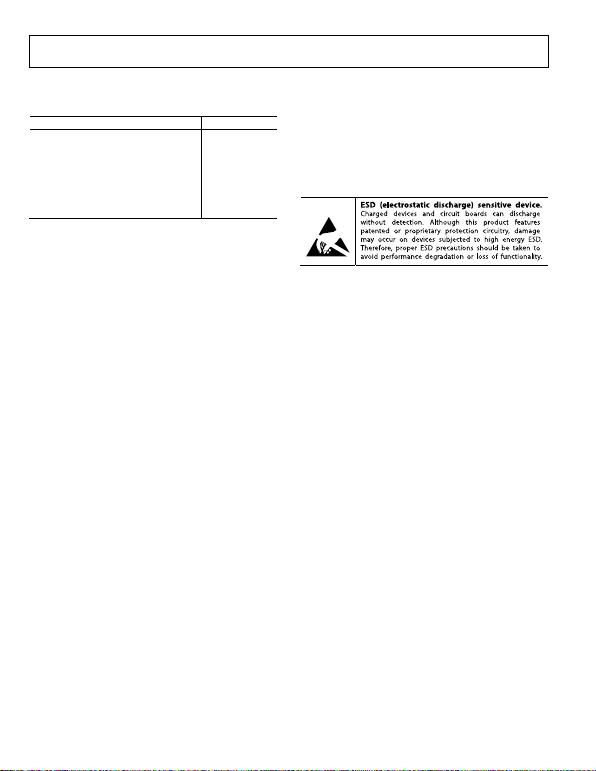Rev. D | Page 4 of 24
ABSOLUTE MAXIMUM RATINGS
Table 2.
Parameter Ratings
Supply 7.5 V
Input Voltage (Pin 1 and Pin 8) VSUPPLY
Storage Temperature Range (N, R) −65°C to +125°C
Ambient Temperature Range, Rated
−40°C to +85°C
(Soldering, 10 sec)
300°C
Stresses above those listed under Absolute Maximum Ratings
can cause permanent damage to the device. This is a stress
rating only; functional operation of the device at these or any
other conditions above those indicated in the operational
section of this specification is not implied. Exposure to absolute
maximum rating conditions for extended periods can affect
device reliability.
ESD CAUTIONRev. D | Page 5 of 24
PIN CONFIGURATION AND FUNCTION DESCRIPTIONS
INP
VPS
ENB
INT
COM
OFS
OUT
INM
TOP VIEW
(Not to Scale)
1
2
3
4
8
7
6
5
01082-002
Figure 2. Pin Configuration
Table 3. Pin Function Descriptions
Pin No. Mnemonic Description
1 INM Signal Input Minus Polarity. Normally at VPOS/2.
2 COM Common Pin (Usually Grounded).
3 OFS Offset Adjustment. External capacitor connection.
4 OUT Logarithmic (RSSI) Output Voltage. ROUT = 12.5 kΩ.
5 INT Intercept Adjustment, ±3 dB. (See the Slope and Intercept Adjustments section.)
6 ENB CMOS-Compatible Chip Enable. Active when high.
7 VPS Positive Supply: 2.7 V to 5.5 V.
8 INP Signal Input Plus Polarity. Normally at VPOS/2. Due to the symmetrical nature of the response, there is no special
significance to the sign of the two input pins. DC resistance from INP to INM = 1.1 kΩ.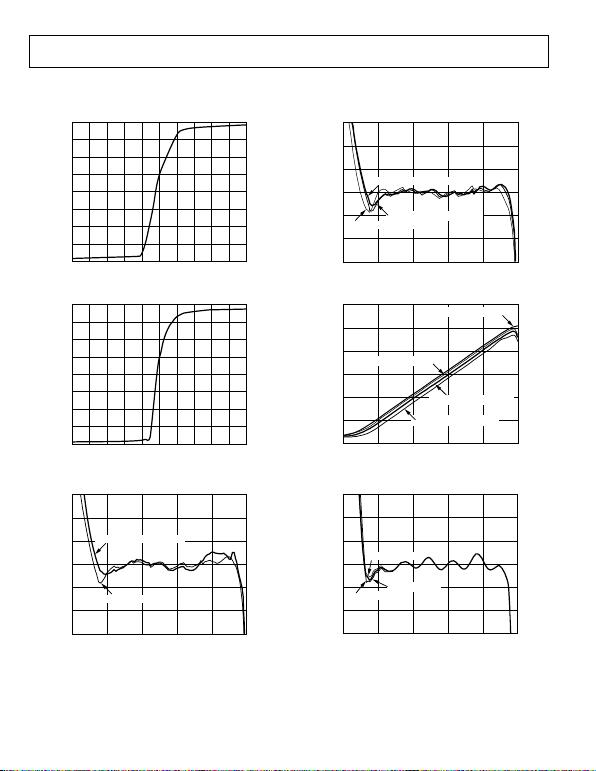Rev. D | Page 6 of 24
TYPICAL PERFORMANCE CHARACTERISTICS
8
3
0
1.0 1.1 1.2 1.3 1.4 1.5 1.6 1.7
7
4
2
1
6
5
1.8 1.9 2.0
01082-003
SUPPLY CURRENT (mA)
VENB (V)
Figure 3. Supply Current vs. VENB (5 V)
8
3
0
1.0 1.1 1.2 1.3 1.4 1.5 1.6 1.7
7
4
2
1
6
5
1.8 1.9 2.0
01082-004
SUPPLY CURRENT (mA)
V
ENB
(V)
Figure 4. Supply Current vs. VENB (3 V)
3
2
–3 200–20–40–60–80
1
0
–1
–2
INPUT FREQUENCY = 100MHz
INPUT FREQUENCY = 300MHz
01082-005
ERROR (dB)
INPUT LEVEL (dBm)
Figure 5. Log Conformance vs. Input Level (dBm), 100 MHz and 300 MHz
3
2
–3 200–20–40–60–80
1
0
–1
–2
01082-006
ERROR (dB)
INPUT LEVEL (dBm)
TEMPERATURE ERROR @ –40°C
TEMPERATURE ERROR @ +25°C
TEMPERATURE ERROR @ +85°C
Figure 6. Log Conformance vs. Input Level (dBm) at −40°C, +25°C, and +85°C
INPUT FREQUENCY 10MHz
INPUT FREQUENCY 100MHz
INPUT FREQUENCY 300MHz
INPUT FREQUENCY 500MHz
3
0200–20–40–60–80
2
1
01082-007
V
OUT
(V)
INPUT LEVEL (dBm)
Figure 7. VOUT vs. Input Level (dBm) at Various Frequencies
CFO VALUE = 0.01µF
CFO VALUE = 1µF
CFO VALUE = 0.1µF
1.5
1.0
–1.5 200–20–40–60–80
0.5
0
–0.5
–1.0
01082-008
ERROR (dB)
INPUT LEVEL (dBm)
Figure 8. Log Conformance vs. CFO Values at 1 kHz Input Frequency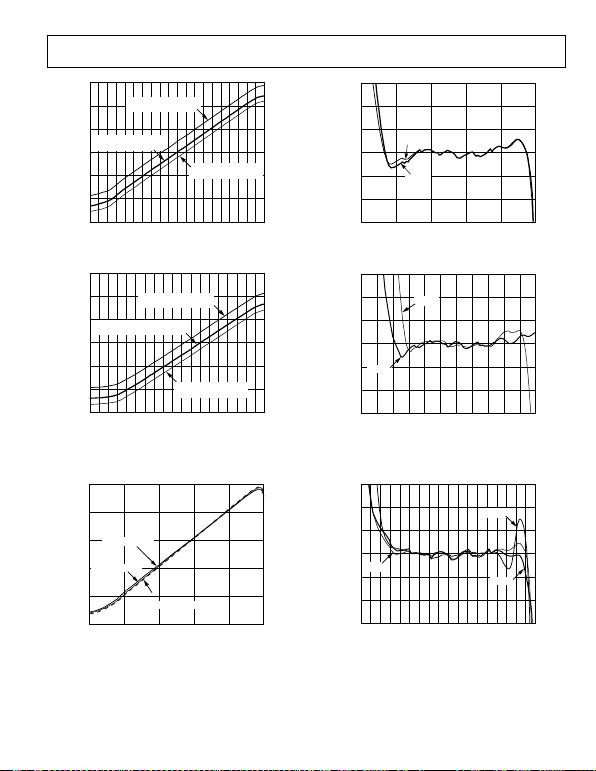Rev. D | Page 7 of 24
3.0
0
0.5
200–20 –10 10–40–60–80 –70 –50 –30
2.0
1.0
2.5
1.5
01082-009
V
OUT
(V)
INPUT LEVEL (dBm)
INT P
IN
= 3.0V
10MHz, INT = –96.52dBm
INT P
IN
= 4.0V
10MHz, INT = –87.71dBm
NO CONNECT ON INT
10MHz, INT = –82.90dBm
Figure 9. VOUT vs. Input Level at 5 V Supply; Showing Intercept Adjustment
3.0
0
0.5
0–20 –10 10–40–60–80 –70 –50 –30
2.0
1.0
2.5
1.5
01082-010
V
OUT
(V)
INPUT LEVEL (dBm)
INT VOLTAGE
INT = 1.0V, INT = –86dBm
INT VOLTAGE
INT NO CONNECT, INT = –71dBm
INT VOLTAGE
INT = 2.0V, INT = –78dBm
Figure 10. VOUT vs. Input Level at 3 V Supply Using AD820 as Buffer,
Gain = +2; Showing Intercept Adjustment
2.5
0200–20–40–60–80
2.0
1.0
1.5
0.5
01082-011
V
OUT
(V)
INPUT LEVEL (dBm)
100MHz @ –40°C
100MHz @ +85°C
100MHz @ +25°C
Figure 11. VOUT vs. Input Level at Three Temperatures (−40°C, +25°C, +85°C)
–INPUT
+INPUT
100MHz
3
2
–3 200–20–40–60–80
1
0
–1
–2
ERROR (dB)
INPUT LEVEL (dBm)
01082-012
Figure 12. Log Conformance vs. Input Level at 100 MHz Showing
Response to Alternative Inputs
3
2
–3 10–10–30–50–70–90
1
0
–1
–2
ERROR (dB)
INPUT LEVEL (dBm)
01082-013
100MHz
500MHz
Figure 13. Log Conformance vs. Input Level at 100 MHz and 500 MHz;
Input Driven Differentially Using Transformer
3
2
–3 100–10–20 20–30–40–50–60–70
1
0
–1
–2
ERROR (dB)
INPUT LEVEL (dBm)
01082-014
100MHz
500MHz
10MHz
Figure 14. Log Conformance vs. Input Level at 3 V Supply
Using AD820 as Buffer, Gain = +2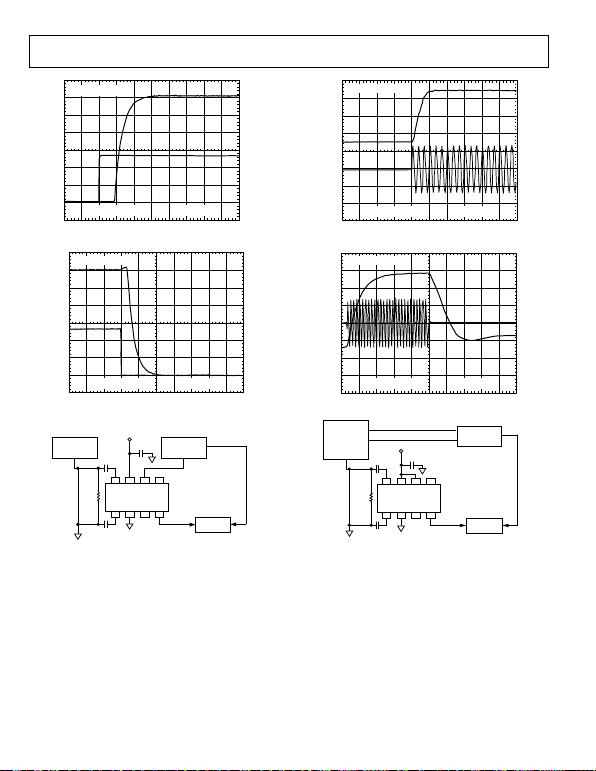Rev. D | Page 8 of 24
V
ENB
CH 2
V
OUT
CH 1
G
ND
01082-015
500ns
CH2 2.00V
CH1 200mV
Figure 15. Power-Up Response Time
V
ENB
CH 2
V
OUT
CH 1
GND
0
1082-016
500ns
CH2 2.00V
CH1 200mV
Figure 16. Power-Down Response Time
0.1µF
V
PS = 5.0V
1nF
1nF
52.3
HP8648B
SIGNAL
GENERATOR
RF OUT OUT
HP8112A
PULSE
GENERATOR
TEK P6139A
10x PROBE
SYNCH OUT
TRIG
NC
INP VPS ENB INT
INM COM OFS OUT
NC
NC = NO CONNECT
8765
234
1
TEK744A
SCOPE
01082-017
Figure 17. Test Setup for Power-Up/Power-Down Response Time
INPUT
SIGNAL
CH2
CH1 GND
2
V
V
OU
T
CH1
CH2
GND
01082-018
200ns
CH2 1.00V
CH1 500mV
Figure 18. VOUT Rise Time
INPUT
SIGNAL
CH2
2.5V
CH1 GND
V
OUT
CH1
CH2
GND
0
1082-019
200ns
CH2 1.00V
CH1 500mV
Figure 19. Large Signal Response Time
PULSE MODE IN
10MHz REF CLK
OUT
EXT TRIG
0.1µF
VPS = 5.0V
1nF
1nF
52.3
HP8648B
SIGNAL
GENERATOR
PULSE
MODULATION
MODE
RF OUT
HP8112A
PULSE
GENERATOR
TEK P6204
FET PROBE
TRIG
OUT
TRIG
NC
INP VPS ENB INT
INM COM OFS OUT
NC
NC = NO CONNECT
8765
234
1
TEK744A
SCOPE
01082-020
Figure 20. Test Setup for VOUT Pulse Response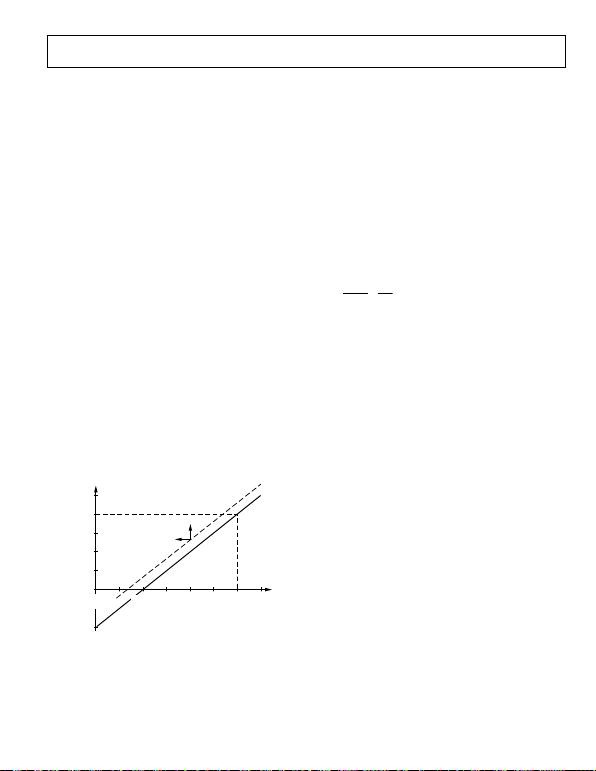Rev. D | Page 9 of 24
LOG AMP THEORY
Logarithmic amplifiers perform a more complex operation than
that of classical linear amplifiers, and their circuitry is significantly
different. A good grasp of what log amps do and how they work
can prevent many pitfalls in their application. The essential purpose
of a log amp is not to amplify, though amplification is utilized to
achieve the function. Rather, it is to compress a signal of wide
dynamic range to its decibel equivalent. It is thus a measurement
device. A better term may be logarithmic converter, because its
basic function is the conversion of a signal from one domain of
representation to another via a precise nonlinear transformation.
Logarithmic compression leads to situations that can be confusing
of a log amp is equivalent to a gain increase ahead of its input.
In the usual case where all the variables are voltages, and regardless
of the particular structure, the relationship between the variables
can be expressed as
)/(log XINY
OUT VVVV = (1)
where:
VOUT is the output voltage.
VY is the slope voltage; the logarithm is usually taken to base 10
(in which case VY is also the volts per decade).
VIN is the input voltage.
VX is the intercept voltage.
All log amps implicitly require two references, in this example,
VX and VY, which determine the scaling of the circuit. The abso-
lute accuracy of a log amp cannot be any better than the accuracy
of its scaling references. Equation 1 is mathematically incomplete
in representing the behavior of a demodulating log amp, such
as the AD8307, where VIN has an alternating sign. However, the
basic principles are unaffected, and this can be safely used as the
starting point in the analyses of log amp scaling.
V
OUT
5V
Y
4V
Y
3V
Y
2V
Y
–2V
Y
V
Y
V
OUT
= 0
V
SHIFT
LOWER INTERCEPT
V
IN
= V
X
0dBc
V
IN
= 10
2
V
X
+40dBc
V
IN
= 10
4
V
X
+80dBc
LOG V
IN
01082-021
V
IN
= 10
–2
V
X
–40dBc
Figure 21. Ideal Log Amp Function
Figure 21 shows the input/output relationship of an ideal log amp,
conforming to Equation 1. The horizontal scale is logarithmic and
spans a wide dynamic range, shown in Figure 21 as over 120 dB, or
at the unique value VIN = VX and ideally becomes negative for
inputs below the intercept. In the ideal case, the straight line
describing VOUT for all values of VIN continues indefinitely in both
directions. The dotted line shows that the effect of adding an
offset voltage, VSHIFT, to the output is to lower the effective intercept
voltage, VX. Exactly the same alteration can be achieved by raising
the gain (or signal level) ahead of the log amp by the factor,
VSHIFT/VY. For example, if VY is 500 mV per decade (25 mV/dB),
an offset of 150 mV added to the output appears to lower the
intercept by two-tenths of a decade, or 6 dB. Adding an offset to
the output is thus indistinguishable from applying an input level
that is 6 dB higher.
The log amp function described by Equation 1 differs from that
of a linear amplifier in that the incremental gain δVOUT/δVIN is a
very strong function of the instantaneous value of VIN, as is
apparent by calculating the derivative. For the case where the
logarithmic base is δ,
IN
Y
IN
OUT
V
V
V
V=
δ
δ
(2)
That is, the incremental gain is inversely proportional to the
instantaneous value of the input voltage. This remains true
for any logarithmic base, which is chosen as 10 for all decibel
related purposes. It follows that a perfect log amp is required to
have infinite gain under classical small signal (zero amplitude)
conditions. Less ideally, this result indicates that whatever
means are used to implement a log amp, accurate response
under small signal conditions (that is, at the lower end of the
dynamic range) demands the provision of a very high gain
bandwidth product. A further consequence of this high gain is
that in the absence of an input signal, even very small amounts
of thermal noise at the input of a log amp cause a finite output
for zero input. This results in the response line curving away
from the ideal shown in Figure 21 toward a finite baseline,
which can be either above or below the intercept. Note that the
value given for this intercept can be an extrapolated value, in
which case the output cannot cross zero, or even reach it, as is
While Equation 1 is fundamentally correct, a simpler formula is
appropriate for specifying the calibration attributes of a log amp
like the AD8307, which demodulates a sine wave input.
VOUT = VSLOPE (PIN – P0) (3)
where:
VOUT is the demodulated and filtered baseband (video or
VSLOPE is the logarithmic slope, now expressed in V/dB (typically
between 15 mV/dB and 30 mV/dB).
PIN is the input power, expressed in decibels relative to some
reference power level.
P0 is the logarithmic intercept, expressed in decibels relative to
the same reference level.
The most widely used reference in RF systems is decibels above
1 mW in 50 , written dBm. Note that the quantity (PIN – P0) is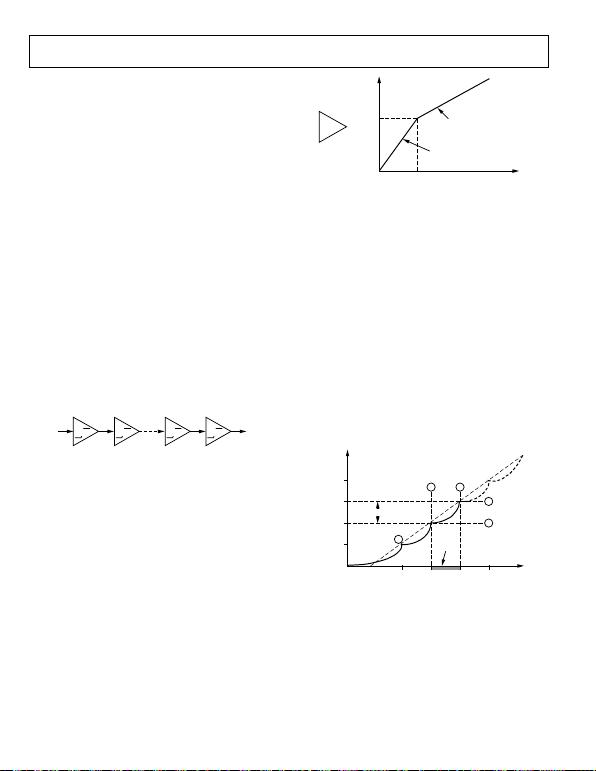Rev. D | Page 10 of 24
just dB. The logarithmic function disappears from the formula
because the conversion has already been implicitly performed
in stating the input in decibels. This is strictly a concession to
popular convention; log amps manifestly do not respond to power
(tacitly, power absorbed at the input), but rather to input voltage.
The use of dBV (decibels with respect to 1 V rms) is more precise,
though still incomplete, because waveform is involved as well.
Because most users think about and specify RF signals in terms
of power, more specifically, in dBm re: 50 , this convention is
used in specifying the performance of the AD8307.
PROGRESSIVE COMPRESSION
Most high speed, high dynamic range log amps use a cascade of
nonlinear amplifier cells (see Figure 22) to generate the logarithmic
function from a series of contiguous segments, a type of piecewise
linear technique. This basic topology immediately opens up the
possibility of enormous gain bandwidth products. For example,
the AD8307 employs six cells in its main signal path, each having
a small signal gain of 14.3 dB (×5.2) and a −3 dB bandwidth of
about 900 MHz. The overall gain is about 20,000 (86 dB) and
the overall bandwidth of the chain is some 500 MHz, resulting
in the incredible gain bandwidth product (GBW) of 10,000 GHz,
about a million times that of a typical op amp. This very high
GBW is an essential prerequisite for accurate operation under
small signal conditions and at high frequencies. In Equation 2,
however, the incremental gain decreases rapidly as VIN increases.
The AD8307 continues to exhibit an essentially logarithmic
response down to inputs as small as 50 V at 500 MHz.
V
X
V
W
STAGE 1 STAGE 2 STAGE N–1 STAGE N
A A A A
0
1082-022
Figure 22. Cascade of Nonlinear Gain Cells
To develop the theory, first consider a scheme slightly different
from that employed in the AD8307, but simpler to explain and
mathematically more straightforward to analyze. This approach
is based on a nonlinear amplifier unit, called an A/1 cell, with
the transfer characteristic shown in Figure 23.
The local small signal gain δVOUT/δVIN is A, maintained for all
inputs up to the knee voltage EK, above which the incremental
gain drops to unity. The function is symmetrical: the same drop
in gain occurs for instantaneous values of VIN less than –EK. The
large signal gain has a value of A for inputs in the range −EK
VIN ≤ +EK, but falls asymptotically toward unity for very large
inputs. In logarithmic amplifiers based on this amplifier function,
both the slope voltage and the intercept voltage must be traceable
to the one reference voltage, EK. Therefore, in this fundamental
analysis, the calibration accuracy of the log amp is dependent
solely on this voltage. In practice, it is possible to separate the
basic references used to determine VY and VX and, in the case of
the AD8307, VY is traceable to an on-chip band gap reference,
whereas VX is derived from the thermal voltage kT/q and is later
temperature corrected.
0
1082-023
SLOPE = A
SLOPE = 1
OUTPUT
AE
K
E
K
0INPUT
A/1
Figure 23. A/1 Amplifier Function
Let the input of an N-cell cascade be VIN, and the final output
be VOUT. For small signals, the overall gain is simply AN. A
six-stage system in which A = 5 (14 dB) has an overall gain
of 15,625 (84 dB). The importance of a very high small signal
gain in implementing the logarithmic function has been noted;
however, this parameter is only of incidental interest in the design
of log amps.
From this point forward, rather than considering gain, analyze
the overall nonlinear behavior of the cascade in response to a
simple dc input, corresponding to the VIN of Equation 1. For
very small inputs, the output from the first cell is V1 = AVIN.
The output from the second cell is V2 = A2 VIN, and so on, up to
VN = AN VIN. At a certain value of VIN, the input to the Nth cell,
VN − 1, is exactly equal to the knee voltage EK. Thus, VOUT = AEK
and because there are N − 1 cells of Gain A ahead of this node,
calculate VIN = EK /AN − 1. This unique situation corresponds to
the lin-log transition (labeled 1 in Figure 24). Below this input,
the cascade of gain cells acts as a simple linear amplifier, whereas
for higher values of VIN, it enters into a series of segments that
lie on a logarithmic approximation (dotted line).
RATIO
OF A
2
1
3
3
2
E
K
/A
N–1
E
K
/A
N–2
E
K
/A
N–3
E
K
/A
N–4
LOG V
IN
(4A–3) E
K
V
OUT
(3A–2) E
K
(2A–1) E
K
AE
K
0
(A–1) E
K
01082-024
Figure 24. First Three Transitions
Continuing this analysis, the next transition occurs when the
input to the N − 1 stage just reaches EK, that is, when VIN =
EK/AN − 2. The output of this stage is then exactly AEK, and it is
easily demonstrated (from the function shown in Figure 23) that
the output of the final stage is (2A − 1)EK (labeled 2 in Figure 24).
Thus, the output has changed by an amount (A − 1)EK for a
change in VIN from EK/AN − 1 to EK/AN − 2, that is, a ratio change of A.
At the next critical point (labeled 3 in Figure 24), the input is
again A times larger and VOUT has increased to (3A − 2)EK, that
is, by another linear increment of (A − 1)EK.Rev. D | Page 11 of 24
Further analysis shows that right up to the point where the input to
the first cell is above the knee voltage, VOUT changes by (A − 1)EK
for a ratio change of A in VIN. This can be expressed as a certain
fraction of a decade, which is simply log10(A). For example,
when A = 5, a transition in the piecewise linear output function
occurs at regular intervals of 0.7 decade (log10(A), or 14 dB
divided by 20 dB). This insight allows the user to immediately
write the volts per decade scaling parameter, which is also the
scaling voltage, VY, when using base 10 logarithms, as
()
)(log
1
10 A
EA
VinChangeLinear
VK
IN
OUT
Y
== (4)
Note that only two design parameters are involved in determining
VY, namely, the cell gain A and the knee voltage, EK, while N,
the number of stages, is unimportant in setting the slope of the
overall function. For A = 5 and EK = 100 mV, the slope would be
a rather awkward 572.3 mV per decade (28.6 mV/dB). A well
designed log amp has rational scaling parameters.
The intercept voltage can be determined by using two pairs of
transition points on the output function (consider Figure 24).
The result is
()
)1/1( +
=AN
K
XA
E
V (5)
For the case under consideration, using N = 6, calculate VZ =
4.28 µV. However, be careful about the interpretation of this
parameter, because it was earlier defined as the input voltage at
which the output passes through zero (see Figure 21). Clearly,
in the absence of noise and offsets, the output of the amplifier
chain shown in Figure 23 can be zero when, and only when,
VIN = 0. This anomaly is due to the finite gain of the cascaded
amplifier, which results in a failure to maintain the logarithmic
approximation below the lin-log transition (labeled 1 in Figure 24).
Closer analysis shows that the voltage given by Equation 5
represents the extrapolated, rather than actual, intercept.
DEMODULATING LOG AMPS
Log amps based on a cascade of A/1 cells are useful in baseband
applications because they do not demodulate their input signal.
However, baseband and demodulating log amps alike can be
made using a different type of amplifier stage, called an A/0 cell.
Its function differs from that of the A/1 cell in that the gain
above the knee voltage EK falls to zero, as shown by the solid
line in Figure 25. This is also known as the limiter function, and
a chain of N such cells are often used to generate hard-limited
output in recovering the signal in FM and PM modes.
01082-025
SLOPE = A
SLOPE = 0
OUTPUT
AEK
0EKINPUT
A/0 tanh
Figure 25. A/0 Amplifier Functions (Ideal and Tanh)
Devices, Inc., communications products incorporating a logarith-
mic IF amplifier all use this technique. It becomes apparent that
the output of the last stage can no longer provide the logarithmic
output because this remains unchanged for all inputs above the
limiting threshold, which occurs at VIN = EK/AN − 1. Instead, the
logarithmic output is now generated by summing the outputs of
all the stages. The full analysis for this type of log amp is only
slightly more complicated than that of the previous case. It is
readily shown that, for practical purposes, the intercept voltage,
VX, is identical to that given in Equation 5, while the slope
voltage is
()
A
AE
VK
Y
10
log
= (6)
Preference for the A/0 style of log amp over one using A/1 cells
stems from several considerations. The first is that an A/0 cell
can be very simple. In the AD8307, it is based on a bipolar
transistor differential pair, having resistive loads, RL, and an
emitter current source, IE. This exhibits an equivalent knee
voltage of EK = 2 kT/q and a small signal gain of A = IERL/EK.
The large signal transfer function is the hyperbolic tangent
(see the dashed line in Figure 25). This function is very precise,
and the deviation from an ideal A/0 form is not detrimental. In
fact, the rounded shoulders of the tanh function result in a
lower ripple in the logarithmic conformance than that obtained
using an ideal A/0 function.
An amplifier composed of these cells is entirely differential
in structure and can thus be rendered very insensitive to
disturbances on the supply lines and, with careful design, to
temperature variations. The output of each gain cell has an
associated transconductance (gm) cell that converts the differen-
tial output voltage of the cell to a pair of differential currents,
which are summed simply by connecting the outputs of all the
gm (detector) stages in parallel. The total current is then converted
back to a voltage by a transresistance stage to generate the
logarithmic output. This scheme is depicted in single-sided
form in Figure 26.Rev. D | Page 12 of 24
01082-026
V
IN
V
LIM
I
OUT
A/0
g
m
A/0
g
m
A/0
g
m
A/0
g
m
g
m
AV
IN
A
2
V
IN
A
3
V
IN
A
4
V
IN
Figure 26. Log Amp Using A/0 Stages and Auxiliary Summing Cells
The chief advantage of this approach is that the slope voltage
can now be decoupled from the knee voltage, EK = 2 kT/q,
which is inherently PTAT. By contrast, the simple summation
of the cell outputs results in a very high temperature coefficient
of the slope voltage given in Equation 6. To do this, the detector
stages are biased with currents (not shown), which are rendered
stable with temperature. These are derived either from the supply
voltage (as in the AD606 and AD608) or from an internal band
affords complete control over the magnitude and temperature
behavior of the logarithmic slope, decoupling it completely
from EK.
A further step is needed to achieve the demodulation response,
required when the log amp converts an alternating input into a
quasi-dc baseband output. This is achieved by altering the gm
cells used for summation purposes to also implement the rectifica-
tion function. Early discrete log amps based on the progressive
compression technique used half-wave rectifiers. This made
postdetection filtering difficult. The AD640 was the first
commercial monolithic log amp to use a full-wave rectifier, a
practice followed in all subsequent Analog Devices types.
These detectors can be modeled as essentially linear gm cells, but
produce an output current independent of the sign of the voltage
applied to the input of each cell; that is, they implement the
absolute value function. Because the output from the later A/0
stages closely approximates an amplitude symmetric square
wave for even moderate input levels (most stages of the amplifier
chain operate in a limiting mode), the current output from
each detector is almost constant over each period of the input.
Somewhat earlier detector stages produce a waveform having
only very brief dropouts, whereas the detectors nearest the
input produce a low level, almost sinusoidal waveform at twice
the input frequency. These aspects of the detector system result
in a signal that is easily filtered, resulting in low residual ripple
on the output.
INTERCEPT CALIBRATION
All monolithic log amps from Analog Devices include accurate
means to position the intercept voltage ,VX (or equivalent power for
a demodulating log amp). Using the scheme shown in Figure 26,
the basic value of the intercept level departs considerably from
that predicted by the simpler analyses given earlier. However,
the intrinsic intercept voltage is still proportional to EK, which is
PTAT (see Equation 5). Recalling that the addition of an offset to
the output produces an effect that is indistinguishable from a
change in the position of the intercept, it is possible to cancel
the left-right motion of VX resulting from the temperature
variation of EK. Do this by adding an offset with the required
temperature behavior.
The precise temperature shaping of the intercept positioning offset
results in a log amp having stable scaling parameters, making it a
true measurement device, for example, as a calibrated received
signal strength indicator (RSSI). In this application, the user is
more interested in the value of the output for an input waveform
that is invariably sinusoidal. Although the input level can alterna-
tively be stated as an equivalent power, in dBm, be sure to work
carefully. It is essential to know the load impedance in which
this power is presumed to be measured.
In RF practice, it is generally safe to assume a reference impedance
of 50  in which 0 dBm (1 mW) corresponds to a sinusoidal ampli-
tude of 316.2 mV (223.6 mV rms). The intercept can likewise be
specified in dBm. For the AD8307, it is positioned at −84 dBm,
corresponding to a sine amplitude of 20 µV. It is important to bear
in mind that log amps do not respond to power, but to the voltage
applied to their input.
The AD8307 presents a nominal input impedance much higher
than 50  (typically 1.1 k low frequencies). A simple input
matching network can considerably improve the sensitivity of
this type of log amp. This increases the voltage applied to the
input and thus alters the intercept. For a 50  match, the voltage
gain is 4.8 and the entire dynamic range moves down by 13.6 dB
(see Figure 35). Note that the effective intercept is a function of
waveform. For example, a square wave input reads 6 dB higher
than a sine wave of the same amplitude and a Gaussian noise
input 0.5 dB higher than a sine wave of the same rms value.
OFFSET CONTROL
In a monolithic log amp, direct coupling between the stages is
used for several reasons. First, this avoids the use of coupling
capacitors, which typically have a chip area equal to that of a
basic gain cell, thus considerably increasing die size. Second, the
capacitor values predetermine the lowest frequency at which the
log amp can operate; for moderate values, this can be as high as
30 MHz, limiting the application range. Third, the parasitic
(backplate) capacitance lowers the bandwidth of the cell, further
limiting the applications.
However, the very high dc gain of a direct-coupled amplifier
raises a practical issue. An offset voltage in the early stages of
the chain is indistinguishable from a real signal. For example,
if it were as high as 400 V, it would be 18 dB larger than the
smallest ac signal (50 V), potentially reducing the dynamic
range by this amount. This problem is averted by using a global
feedback path from the last stage to the first, which corrects this
offset in a similar fashion to the dc negative feedback applied
around an op amp. The high frequency components of the
signal must be removed to prevent a reduction of the HF gain in
the forward path.Rev. D | Page 13 of 24
In the AD8307, this is achieved by an on-chip filter, providing
sufficient suppression of HF feedback to allow operation above
1 MHz. To extend the range below this frequency, an external
capacitor can be added. This permits the high-pass corner to be
lowered to audio frequencies using a capacitor of modest value.
Note that this capacitor has no effect on the minimum signal
frequency for input levels above the offset voltage; this extends
down to dc (for a signal applied directly to the input pins). The
offset voltage varies from part to part; some exhibit essentially
stable offsets of under 100 V without the benefit of an offset
EXTENSION OF RANGE
The theoretical dynamic range for the basic log amp shown in
Figure 26 is AN. For A = 5.2 (14.3 dB) and N = 6, it is 20,000 or
86 dB. The actual lower end of the dynamic range is largely
determined by the thermal noise floor, measured at the input of
the chain of amplifiers. The upper end of the range is extended
upward by the addition of top-end detectors. The input signal is
applied to a tapped attenuator, and progressively smaller signals
are applied to three passive rectifying gm cells whose outputs are
summed with those of the main detectors. With care in design,
the extension to the dynamic range can be seamless over the full
frequency range. For the AD8307, it amounts to a further 27 dB.
Therefore, the total dynamic range is theoretically 113 dB.
The specified range of 90 dB (−74 dBm to +16 dBm) is for high
accuracy and calibrated operation, and includes the low end
degradation due to thermal noise and the top end reduction due
to voltage limitations. The additional stages are not redundant, but
are needed to maintain accurate logarithmic conformance over
the central region of the dynamic range, and in extending the
usable range considerably beyond the specified range. In
applications where log conformance is less demanding, the
AD8307 can provide over 95 dB of range.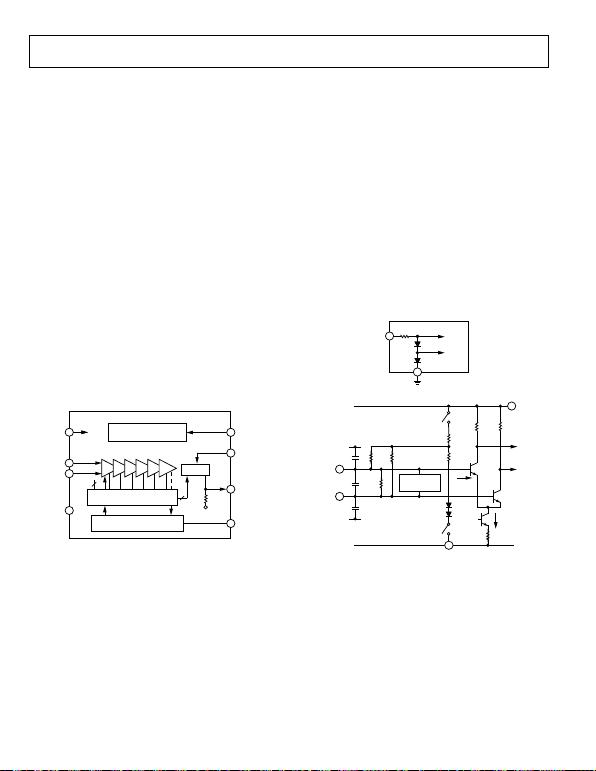Rev. D | Page 14 of 24
INTERFACES
The AD8307 comprises six main amplifier/limiter stages, each
having a gain of 14.3 dB and small signal bandwidth of 900 MHz;
the overall gain is 86 dB with a −3 dB bandwidth of 500 MHz.
These six cells and their associated gm styled full-wave detectors
handle the lower two-thirds of the dynamic range. Three top-
end detectors, placed at 14.3 dB taps on a passive attenuator,
handle the upper third of the 90 dB range. Biasing for these cells
is provided by two references: one determines their gain and the
other is a band gap circuit that determines the logarithmic slope
and stabilizes it against supply and temperature variations. The
AD8307 can be enabled or disabled by a CMOS-compatible level
at ENB (Pin 6). The first amplifier stage provides a low voltage
noise spectral density (1.5 nV/√Hz).
The differential current-mode outputs of the nine detectors are
summed and then converted to single-sided form in the output
stage, nominally scaled 2 A/dB. The logarithmic output voltage
is developed by applying this current to an on-chip 12.5 kΩ
resistor, resulting in a logarithmic slope of 25 mV/dB (that is,
500 mV/decade) at Pin OUT. This voltage is not buffered, allowing
the use of a variety of special output interfaces, including the
addition of postdemodulation filtering. The last detector stage
which is accurately positioned to make optimal use of the full
output voltage range available. The intercept can be adjusted
using the INT pin, which adds or subtracts a small current to
the signal current.
01082-027
BAND GAP REFERENCE
AND BIASING
SIX 14.3dB 900MHz
AMPLIFIER STAGES
MIRROR
INPUT-OFFSET
COMPENSATION LOOP
C
OM
INM
INP
ENBVPS
INT
OUT
OFS
7.5mA
1.1k
3
2
2µA
/dB
12.5k
COM
NINE DETECTOR CELLS
SPACED 14.3dB
–INP
+INP
8
1
2
7
5
6
4
3
Figure 27. Main Features of the AD8307
The last gain stage also includes an offset sensing cell. This
generates a bipolarity output current when the main signal path
has an imbalance due to accumulated dc offsets. This current is
integrated by an on-chip capacitor, which can be increased in
value by an off-chip component at OFS. The resulting voltage is
used to null the offset at the output of the first stage. Because it
does not involve the signal input connections, whose ac-coupling
capacitors otherwise introduce a second pole in the feedback
path, the stability of the offset correction loop is assured.
complementary bipolar process. Most resistors are thin film
types having a low temperature coefficient of resistance (TCR)
and high linearity under large signal conditions. Their absolute
tolerance is typically within ±20%. Similarly, the capacitors have
a typical tolerance of ±15% and essentially zero temperature or
voltage sensitivity. Most interfaces have additional small junction
capacitances associated with them due to active devices or ESD
protection; these can be neither accurate nor stable. Component
numbering in each of these interface diagrams is local.
ENABLE INTERFACE
The chip enable interface is shown in Figure 28. The currents in
the diode-connected transistors control the turn-on and turn-
off states of the band gap reference and the bias generator, and
are a maximum of 100 A when Pin 6 is taken to 5 V, under
worst-case conditions. Left unconnected, or at a voltage below
1 V, the AD8307 is disabled and consumes a sleep current of
under 50 A; tied to the supply, or at a voltage above 2 V, it is
fully enabled. The internal bias circuitry is very fast, typically
<100 ns for either off or on. In practice, the latency period
before the log amp exhibits its full dynamic range is more likely
to be limited by factors relating to the use of ac coupling at the
input or the settling of the offset control loop.
COM
ENB
40k
TO BIAS
STAGES
6
2
01082-028
Figure 28. Enable Interface
TOP-END
DETECTORS
COM
INP
INM
C
D
C
P
C
M
COM
4k~3k
125125
6k
6k
2k2k
TYP 2.2V FOR
3V SUPPLY,
3.2V AT 5V
S
S
PS
COM
I
E
2.4mA
Q1
Q2
01082-029
2
7
8
1
Figure 29. Signal Input Interface
INPUT INTERFACE
Figure 29 shows the essentials of the signal input interface.
CP and CM are the parasitic capacitances to ground; CD is the
differential input capacitance, mostly due to Q1 and Q2. In
most applications, both input pins are ac-coupled. The switches
close when ENB is asserted. When disabled, the inputs float,
bias current IE is shut off, and the coupling capacitors remain
charged. If the log amp is disabled for long periods, small leakage
currents discharge these capacitors. If they are poorly matched,
charging currents at power-up can generate a transient input
voltage that can block the lower reaches of the dynamic range
until it has become much less than the signal.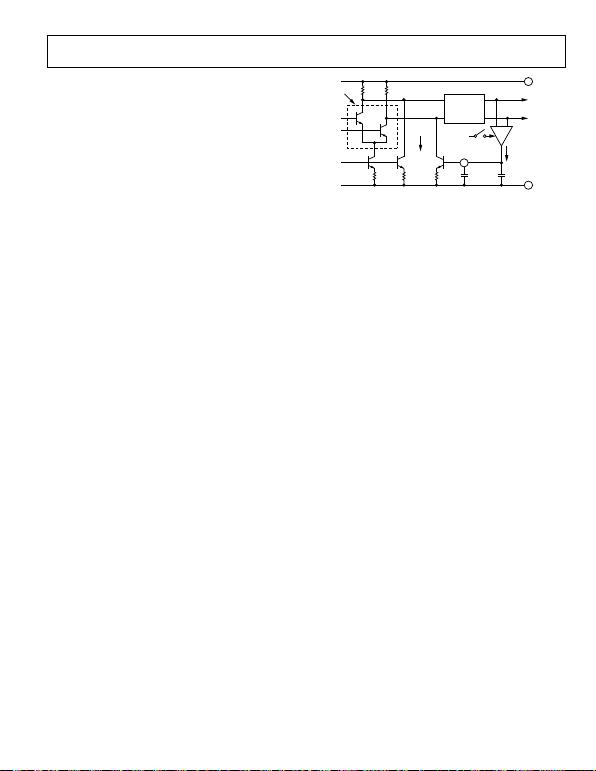Rev. D | Page 15 of 24
In most applications, the signal is single sided and can be applied
to either Pin 1 or Pin 8, with the other pin ac-coupled to ground.
Under these conditions, the largest input signal that can be
handled by the AD8307 is 10 dBm (sine amplitude of ±1 V)
when operating from a 3 V supply; 16 dBm can be handled
using a 5 V supply. The full 16 dBm can be achieved for supplies
down to 2.7 V, using a fully balanced drive. For frequencies
above about 10 MHz, this is most easily achieved using a matching
network. Using such a network, having an inductor at the input,
the input transient is eliminated. Occasionally, it is desirable to
use the dc-coupled potential of the AD8307. The main challenge
is to present signals to the log amp at the elevated common-mode
input level, requiring the use of low noise, low offset buffer
amplifiers. Using dual supplies of ±3 V, the input pins can
operate at ground potential.
OFFSET INTERFACE
The input-referred dc offsets in the signal path are nulled via
the interface associated with Pin 3, shown in Figure 30. Q1 and
Q2 are the first stage input transistors, with their corresponding
load resistors (125 ). Q3 and Q4 generate small currents, which
can introduce a dc offset into the signal path. When the voltage
on OFS is at about 1.5 V, these currents are equal and nominally
64 A. When OFS is taken to ground, Q4 is off and the effect of the
current in Q3 is to generate an offset voltage of 64 V × 125  =
8 mV. Because the first stage gain is ×5, this is equivalent to an
input offset (INP to INM) of 1.6 mV. When OFS is taken to its
most positive value, the input-referred offset is reversed to −1.6 mV.
If true dc coupling is needed, down to very small inputs, this auto-
matic loop must be disabled and the residual offset eliminated
In normal operation, however, using an ac-coupled input signal,
the OFS pin should be left open. Any residual input offset voltage
is then automatically nulled by the action of the feedback loop.
The gm cell, which is gated off when the chip is disabled, converts
any output offset (sensed at a point near the end of the cascade
of amplifiers) to a current. This is integrated by the on-chip
capacitor, CHP, and any added external capacitance, COFS, to
generate an error voltage, which is applied back to the input
stage in the polarity needed to null the output offset. From a
small signal perspective, this feedback alters the response of the
amplifier, which, rather than behaving as a fully dc-coupled
system, now exhibits a zero in its ac transfer function, resulting
in a closed-loop high-pass corner at about 1.5 MHz.
48k
125125
MAIN GAIN
STAGES
Q2
Q1
Q3
64µA AT
BALANCE
Q4
S
AVERAGE
ERROR
CURRENT
OFS
TO LAST
DETECTOR
C
OFS
C
HP
COM
VPS
36k
INPUT
STAGE
01082-030
BIAS, ~1.2V
2
3
7
g
m
Figure 30. Offset Interface and Offset Nulling Path
The offset feedback is limited to a range of ±1.6 mV; signals larger
than this override the offset control loop, which only affects perfor-
mance for very small inputs. An external capacitor reduces the
high-pass corner to arbitrarily low frequencies; using 1 F, this
corner is below 10 Hz. All Analog Devices log amps use an offset
nulling loop; the AD8307 differs in using this single-sided form.
OUTPUT INTERFACE
The outputs from the nine detectors are differential currents,
having an average value that is dependent on the signal input
level, plus a fluctuation at twice the input frequency. The currents
are summed at Node LGP and Node LGM in Figure 31. Further
currents are added at these nodes, to position the intercept, by
slightly raising the output for zero input, and to provide tempera-
ture compensation. Because the AD8307 is not laser trimmed,
there is a small uncertainty in both the log slope and the log
intercept. These scaling parameters can be adjusted.
For zero signal conditions, all the detector output currents are
equal. For a finite input of either polarity, their difference is
converted by the output interface to a single-sided unipolar
current nominally scaled 2 A/dB (40 A/decade) at Pin OUT.
An on-chip 12.5 k resistor, R1, converts this current to a voltage
of 25 mV/dB. C1 and C2 are effectively in shunt with R1 and form
a low-pass filter pole with a corner frequency of about 5 MHz.
The pulse response settles to within 1% of the final value within
300 ns. This integral low-pass filter provides adequate smoothing
in many IF applications. At 10.7 MHz, the 2f ripple is 12.5 mV
in amplitude, equivalent to ±0.5 dB, and only 0.5 mV (±0.02 dB)
at f = 50 MHz. A filter capacitor, CFLT, added from Pin OUT to
ground lowers this corner frequency. Using 1 F, the ripple is
maintained to less than ±0.5 dB down to input frequencies of
100 Hz. Note that COFS should also be increased in low frequency
applications, and is typically made equal to CFLT.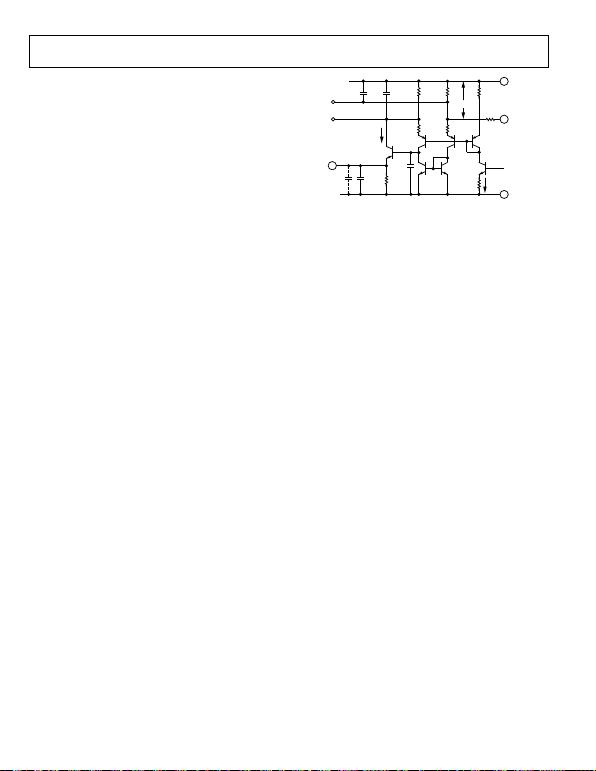Rev. D | Page 16 of 24
It can be desirable to increase the speed of the output response,
with the penalty of increased ripple. One way to do this is by
connecting a shunt load resistor from Pin OUT to ground,
which raises the low-pass corner frequency. This also alters the
logarithmic slope, for example, to 7.5 mV/dB using a 5.36 k
resistor, while reducing the 10% to 90% rise time to 25 ns. The
ripple amplitude for the 50 MHz input remains at 0.5 mV, but
this is now equivalent to ±0.07 dB. If a negative supply is available,
the output pin can be connected directly to the summing node
of an external op amp connected as an inverting mode transresis-
tance stage.
Note that while the AD8307 can operate down to supply voltages
of 2.7 V, the output voltage limit is reduced when the supply
drops below 4 V. This characteristic is the result of necessary
headroom requirements, approximately two VBE drops, in the
design of the output stage.
1.25k
25mV/dB
OUT
2µA/dB
0µA TO 220µA
INT
FROM ALL
DETECTORS
C
FLT
C2
1pF
VPS
BIAS
1.25k1.25k
~400mV
8.25k
60k
60µA
COM
R1
12.5k
C1
2.5pF
3pF
LGP
LGM
1.25k
01082-031
4
7
5
2
Figure 31. Simplified Output Interface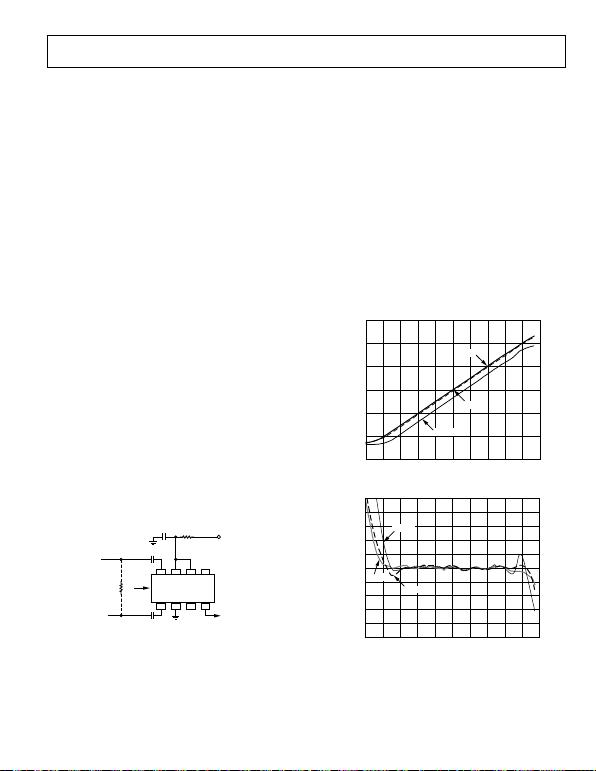Rev. D | Page 17 of 24
THEORY OF OPERATION
The AD8307 has very high gain and a bandwidth from dc to
over 1 GHz, at which frequency the gain of the main path is
still over 60 dB. Consequently, it is susceptible to all signals
within this very broad frequency range that find their way to
the input terminals. It is important to remember that these are
indistinguishable from the wanted signal, and has the effect
of raising the apparent noise floor (that is, lowering the useful
dynamic range). For example, while the signal of interest can
be an IF of 50 MHz, any of the following could easily be larger
than the IF signal at the lower extremities of its dynamic range:
60 Hz hum (picked up due to poor grounding techniques),
spurious coupling (from a digital clock source on the same PC
board), and local radio stations, for example.
Careful shielding is essential. A ground plane should be used to
provide a low impedance connection to the common pin, COM,
for the decoupling capacitors used at VPS, and as the output
ground. It is inadvisable to assume that the ground plane is
equipotential. Neither of the inputs should be ac-coupled directly
to the ground plane, but should be kept separate from it, being
returned instead to the low associated with the source. This can
mean isolating the low side of an input connector with a small
resistance to the ground plane.
BASIC CONNECTIONS
Figure 32 shows the simple connections suitable for many
applications. The inputs are ac coupled by C1 and C2, which
should have the same value, for example, CC. The coupling time
constant is RIN CC/2, thus forming a high-pass corner with a
3 dB attenuation at fHP = 1/(pRINCC ). In high frequency applica-
tions, fHP should be as large as possible to minimize the coupling
of unwanted low frequency signals. Conversely, in low frequency
applications, a simple RC network forming a low-pass filter
should be added at the input for the same reason. For the case
where the generator is not terminated, the signal range should
be expressed in terms of the voltage response and should extend
from −85 dBV to +6 dBV.
C2 = C
C
C1 = C
C
OUTPUT
25mV/dB
01082-032
R
T
INPUT
–75dBm TO
+16dBm
NC
INP VPS ENB INT
INM COM OFS OUT
NC
NC = NO CONNECT
8765
234
1
1.1k
4.7
0.1µ
F
R
IN
V
P
, 2.7V TO 5.5
V
AT ~8mA
Figure 32. Basic Connections
Where it is necessary to terminate the source at a low impedance,
the resistor RT should be added, with allowance for the shunting
effect of the basic 1.1 k input resistance (RIN) of the AD8307.
For example, to terminate a 50  source, a 52.3  1% tolerance
resistor should be used. This can be placed on the input side or
the log amp side of the coupling capacitors; in the former case,
smaller capacitors can be used for a given frequency range; in
the latter case, the effective RIN is lowered directly at the log
amp inputs.
Figure 33 shows the output vs. the input level, in dBm, when
driven from a terminated 50  generator, for sine inputs at
10 MHz, 100 MHz, and 500 MHz; Figure 34 shows the typical
logarithmic conformance under the same conditions. Note that
10 dBm corresponds to a sine amplitude of 1 V, equivalent to an
rms power of 10 mW in a 50  termination. However, if the
termination resistor is omitted, the input power is negligible.
The use of dBm to define input level therefore needs to be
considered carefully in connection with the AD8307.
3.0
2.5
0
2.0
1.5
1.0
0.5 500MHz
100MHz
10MHz
01082-033
–80 –70 –60 –50 –40 –30 –20 –10 0 10 20
OUTPUT VOLTAGE (V)
INPUT LEVEL (dBm)
Figure 33. Log Response at 10 MHz, 100 MHz, and 500 MHz
5
4
3
2
1
–4
–5
500MHz
100MHz
10MHz
–3
–2
–1
0
01082-034
–80 –70 –60 –50 –40 –30 –20 –10 0 10 20
ERROR (dB)
INPUT LEVEL (dBm)
Figure 34. Logarithmic Law Conformance at 10 MHz, 100 MHz, and 500 MHz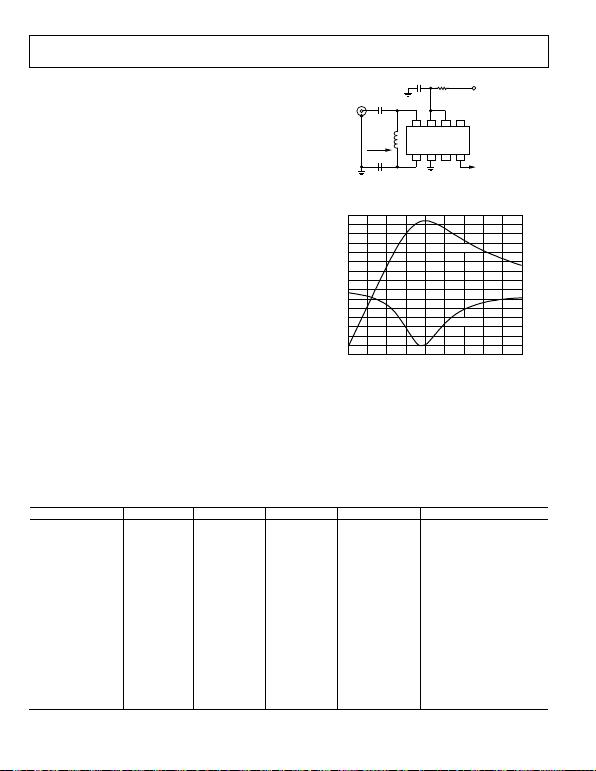Rev. D | Page 18 of 24
INPUT MATCHING
Where higher sensitivity is required, an input matching network
is valuable. Using a transformer to achieve the impedance
transformation also eliminates the need for coupling capacitors,
which lowers the offset voltage generated directly at the input,
and balances the drives to Pin INP and Pin INM. The choice of
turns ratio depends somewhat on the frequency. At frequencies
below 50 MHz, the reactance of the input capacitance is much
higher than the real part of the input impedance. In this frequency
range, a turns ratio of about 1:4.8 lowers the input impedance to
50  while raising the input voltage, thus lowering the effect of
the short-circuit noise voltage by the same factor. There is a
small contribution from the input noise current, so the total
noise is reduced by a lesser factor. The intercept is also lowered
by the turns ratio; for a 50  match, it is reduced by 20 log10
(4.8) or 13.6 dB.
NARROW-BAND MATCHING
Transformer coupling is useful in broadband applications. How-
ever, a magnetically coupled transformer may not be convenient in
some situations. At high frequencies, it is often preferable to use
a narrow-band matching network, as shown in Figure 35.
Using a narrow-band matching network has several advantages.
The same voltage gain is achieved, providing increased sensitivity,
but a measure of selectivity is also introduced. The component
count is low: two capacitors and an inexpensive chip inductor.
Further, by making these capacitors unequal, the amplitudes at
Pin INP and Pin INM can be equalized when driving from a
single-sided source, that is, the network also serves as a balun.
Figure 36 shows the response for a center frequency of 100 MHz.
Note the very high attenuation at low frequencies. The high fre-
quency attenuation is due to the input capacitance of the log amp.
C2
C1
OUTPUT
25mV/dB
01082-035
50 INPUT
–88dBm TO
+3dBm
NC
INP VPS ENB INT
INM COM OFS OUT
NC
NC = NO CONNECT
Z
IN
= 50
8765
234
1
4.7
0.1µ
F
L
M
V
P
, 2.7V TO 5.5
V
AT ~8mA
Figure 35. High Frequency Input Matching Network
14
4
–1
3
2
1
0
INPUT
GAIN
9
8
7
6
5
13
12
11
10
01082-036
DECIBELS
FREQUENCY (MHz)
60 150140130120110100908070
Figure 36. Response of 100 MHz Matching Network
Table 4 provides solutions for a variety of center frequencies (fC)
and matching impedances (ZIN) of nominally 50  and 100 .
The unequal capacitor values were chosen to provide a well-
balanced differential drive and to allow better centering of the
frequency response peak when using standard value components,
which generally results in a ZIN that is not exact. The full AD8307
HF input impedance and the inductor losses are included in the
modeling.
Table 4. Narrow-Band Matching Values
fC (MHz) ZIN (Ω) C1 (pF) C2 (pF) LM (nH) Voltage Gain (dB)
10 45 160 150 3300 13.3
20 44 82 75 1600 13.4
50 46 30 27 680 13.4
100 50 15 13 330 13.4
150 57 10 8.2 220 13.2
200 57 7.5 6.8 150 12.8
250 50 6.2 5.6 100 12.3
500 54 3.9 3.3 39 10.9
10 103 100 91 5600 10.4
20 102 51 43 2700 10.4
50 99 22 18 1000 10.6
100 98 11 9.1 430 10.5
150 101 7.5 6.2 260 10.3
200 95 5.6 4.7 180 10.3
250 92 4.3 3.9 130 9.9
500 114 2.2 2.0 47 6.8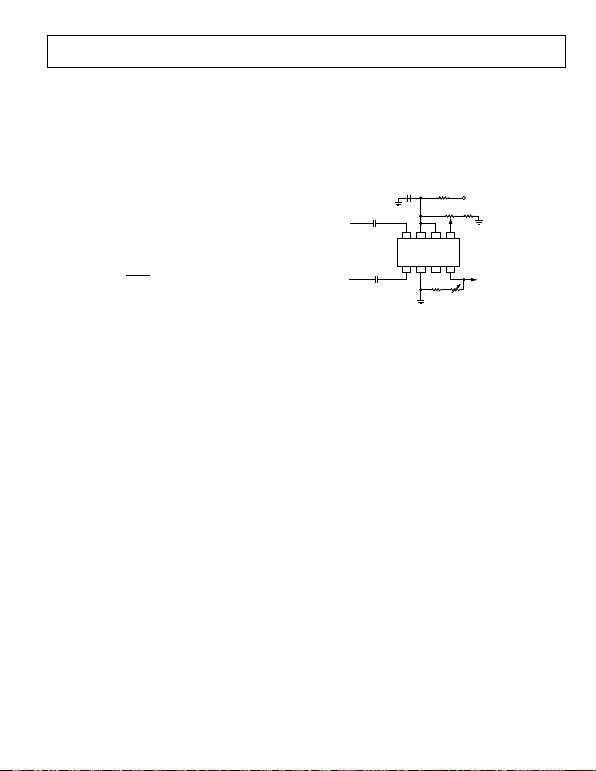Rev. D | Page 19 of 24
Where higher calibration accuracy is needed, the adjustments
shown in Figure 37 can be used, either singly or in combination.
The log slope is lowered to 20 mV/dB by shunting the nominally
12.5 k on-chip load resistor (see Figure 31) with 50 k, adjusted
by VR1. The calibration range is ±10% (18 mV/dB to 22 mV/dB),
including full allowance for the variability in the value of the
two input levels, provided by an accurate signal generator, spaced
over the central portion of the log amps dynamic range, for
example, −60 dBm and 0 dBm. An AM modulated signal at the
center of the dynamic range can also be used. For a modulation
depth, M, expressed as a fraction, the decibel range between the
peaks and troughs over one cycle of the modulation period is
given by
M
M
dB
+
=Δ 1
1
log20 10 (7)
For example, using an rms signal level of −40 dBm with a 70%
modulation depth (M = 0.7), the decibel range is 15 dB, as the
signal varies from −47.5 dBm to −32.5 dBm.
sufficient to absorb the worst-case intercept error in the AD8307,
plus some system level errors. For greater range, set RS to zero.
VR2 is adjusted while applying an accurately known CW signal
near the lower end of the dynamic range to minimize the effect
of any residual uncertainty in the slope. For example, to position
the intercept to −80 dBm, a test level of −65 dBm can be applied
and VR2 adjusted to produce a dc output of 15 dB above zero at
25 mV/dB, which is 0.3 V.
01082-037
C2 = C
C
C1 = C
C
20mV/dB
±10%
INPUT
–75dBm TO
+16dBm
INP VPS ENB INT
INM COM OFS OUT
NC
NC = NO CONNECT
8765
234
1
4.7
VR2
50kR
S
0.1µ
F
V
P
, 2.7V TO 5.5V
AT ~8mA
±3dB
VR1
50k
32.4k
FOR V
P
= 3V, R
S
= 20k
V
P
= 5V, R
S
= 51k
Figure 37. Slope and Intercept Adjustments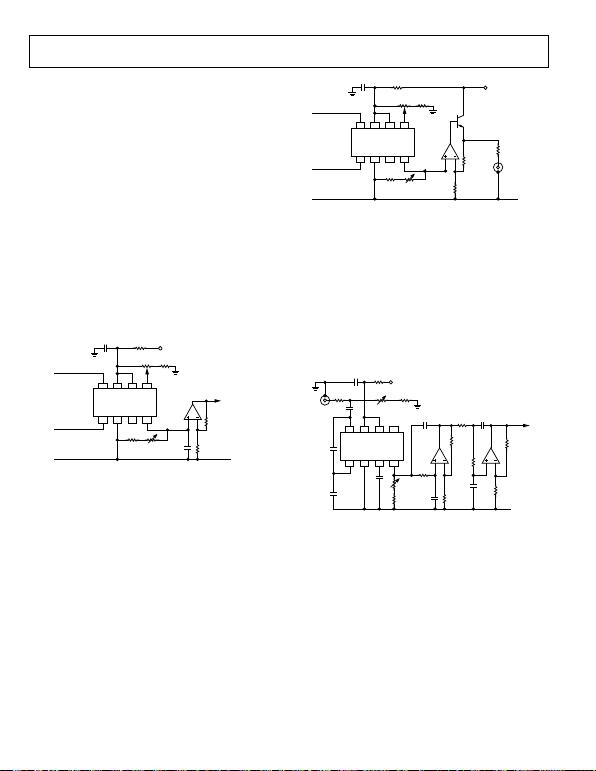Rev. D | Page 20 of 24
APPLICATIONS INFORMATION
The AD8307 is a highly versatile and easily applied log amp
requiring very few external components. Most applications of this
device can be accommodated using the simple connections shown
in the preceding section.
BUFFERED OUTPUT
The output can be buffered and the slope optionally increased by
using an op amp. If the single-supply capability is to be preserved,
capable of operating from a 2.7 V supply and features a rail-to-
rail output capability; it is available in a 5-lead version and in
dual form as the 8-lead AD8032. Figure 38 shows how the slope
can be increased to 50 mV/dB (1 V per decade), requiring a 5 V
supply (90 dB times 50 mV is a 4.5 V swing). VR1 provides a
±10% slope adjustment; VR2 provides a ±3 dB intercept range.
With R2 = 4.99 k, the slope is adjustable to 25 mV/dB, allowing
the use of a 2.7 V supply. Setting R2 to 80.6 k, it is raised to
100 mV/dB, providing direct reading in decibels on a digital
voltmeter. Because a 90 dB range now corresponds to a 9 V swing,
a supply of at least this amount is needed for the op amp.
20mV/dB
01082-038
OUTPUT
50mV/dB
±10%
INPUT
75dBm TO
+16dBm
INP VPS ENB INT
INM COM OFS OUT
NC
NC = NO CONNECT
8765
234
1
4.7
VR2
50k
R2
30.1k
COM
R1
20k
R
S
0.1µF
V
P
, 2.7V TO 5.5V
±3dB
VR1
50k
32.4kC1
FOR V
P
= 3V, R
S
= 20k
V
P
= 5V, R
S
= 51k
Figure 38. Log Amp with Buffered Output
C1 is optional; it lowers the corner frequency of the low-pass
output filter. A value of 0.1 F should be used for applications in
which the output is measured on a voltmeter or other low speed
device. On the other hand, when C1 is omitted, the 10% to 90%
response time is under 200 ns and is typically 300 ns to 99% of
the final value. To achieve faster response times, it is necessary
to lower the load resistance at the output of the AD8307, then
restore the scale using a higher gain in the op amp. Using 8.33 k,
the basic slope is 10 mV/dB; this can be restored to 25 mV/dB
using a buffer gain of 2.5. The overall 10% to 90% response time
is under 100 ns. Figure 39 shows how the output current capability
can be augmented to drive a 50  load; RT optionally provides
reverse termination, which halves the slope to 12.5 mV/dB.
FOUR-POLE FILTER
In low frequency applications, for example, audio down to
20 Hz, it is useful to employ the buffer amplifier as a multipole
low-pass filter to achieve low output ripple while maintaining a
rapid response time to changes in signal level.
25mV/dB
2N3904
10mV/dB
±18%
01082-039
OUTPUT
50
MINIMUM
INPUT
–75dBm TO
+16dBm
INP VPS ENB INT
INM COM OFS OUT
NC
NC = NO CONNECT
8765
234
1
4.7
VR2
50k
R2
3.01k
R1
2k
RS
0.1µ
F
VP, 2.7V TO 5.5V
±3dB
VR1
5k
6.34k
RT
(OPTIONAL)
COM
Figure 39. Cable Driving Log Amp
In Figure 40, the capacitor values are chosen for operation in the
audio field, providing a corner frequency of 10 Hz, an attenuation
of 80 dB/decade above this frequency, and a 1% settling time of
150 ms (0.1% in 175 ms). The residual ripple is 4 mV (±0.02 dB)
when the input to the AD8307 is at 20 Hz. This filter can easily
be adapted to other frequencies by proportional scaling of C5 to C7
(for example, for 100 kHz use 100 pF). Placed ahead of a digital
multimeter, the convenient slope scaling of 100 mV/dB requires
only a repositioning of the decimal point to read directly in
decibels. The supply voltage for the filter must be large enough to
support the dynamic range; a minimum of 9 V is needed for most
applications; 12 V is recommended.
VR2
50k
SLOPE
01082-040
OUTPUT
100mV/dB
INPUT 5m
V
T
O 160V rms
INP VPS ENB INT
INM COM OFS OUT
8765
234
1
4.7
VR1
2k
NC
R1
50k
93k
80.6k
32.4k
34k
422
0.1µF
V
P
C6
1µF
75k
C7
1µF
C2
10µF
C3
2.5nF
+
+
C1
10µF
C1 TO C8 AS NEEDED.
NOTE POLARITIES IF TANTALUM
CAPACITORS ARE USED.
C4
1µF
+
+
+
+
C8
1µF
7.32k
100k
34k
+
C5
1µF
INT ±4dB
COM
Figure 40. Log Amp with Four-Pole Low-Pass Filter
Figure 40 also shows the use of an input attenuator that can
optionally be employed to produce a useful wide range ac
voltmeter with direct decibel scaling. The basic range of −73
dBm to +17 dBm (that is, 50 V rms to 1.6 V rms, for sine
excitations) is shifted for illustrative purposes to 5 mV to 160 V
rms (at which point the power in R1 is 512 mW). Because the
basic input resistance of the AD8307 is not precise, VR1 is used
to center the signal range at its input, doubling as a ±4 dB intercept
adjustment. The low frequency response extends to 15 Hz; a
higher corner frequency can be selected as needed by scaling C1
and C2. The shunt capacitor, C3, is used to lower the high
frequency bandwidth
to about 100 kHz, and thus lower the susceptibility to spurious
signals. Other values should be chosen as needed for the coupling
and filter capacitors.